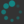## Exercises

1. Compute $\cos$ and $\sin$ of $30^\circ$ and $60^\circ.$
2. Define the tangent function by $$\tan\theta \Defeq \dfrac{\sin\theta}{\cos\theta};$$ that is, $\tan\theta$ is the slope of the line passing through the origin at angle $\theta.$ We define $\tan90^\circ = \tan270^\circ = \infty;$ while we will later encounter the symbols $+\infty$ and $-\infty,$ this is an unsigned or projective infinity.

Compute $\tan\theta$ for the standard angles $\theta=0^\circ,\, 30^\circ,\, 45^\circ,\, 60^\circ,\, 90^\circ.$

3. Write down $\cos,$ $\sin,$ and $\tan$ of the standard angles in every quadrant of the unit circle.
4. Consider the following diagram: By calculating the length of the green line segment in two different ways, establish the identity $\begin{equation} \displaystyle\label{silly-formula} 2\sin\left(\frac{\alpha-\beta}2\right) = \pm\sqrt{(\cos\alpha - \cos\beta)^2 + (\sin\alpha - \sin\beta)^2} \end{equation}$
5. Once we've established \eqref{silly-formula}, we can derive all the usual trigonometric identities using algebraic manipulations, with no further geometric insight required.
1. Use \eqref{silly-formula} to derive the "half-angle formulas" \begin{align} \cos(\theta/2) &= \pm\sqrt{\frac{1+\cos(\theta)}2} \label{half-cos}\\ \sin(\theta/2) &= \pm\sqrt{\frac{1-\cos(\theta)}2}. \label{half-sin} \end{align}
2. Use \eqref{half-cos} and \eqref{half-sin} to derive the "double-angle formulas" \begin{align*} \cos(2\theta) &= \cos^2(\theta) - \sin^2(\theta)\\ &= 2\cos^2(\theta) - 1\\ &= 1 - 2\sin^2(\theta)\\ \sin(2\theta) &= 2\cos(\theta)\sin(\theta) \end{align*}
3. Derive the "angle-difference" formulas \begin{align} \label{cos-diff} \cos(\alpha-\beta) &= \cos(\alpha)\cos(\beta) + \sin(\alpha)\sin(\beta)\\ \label{sin-diff} \sin(\alpha-\beta) &= \sin(\alpha)\cos(\beta) - \cos(\alpha)\sin(\beta) \end{align} and the "angle-sum" formulas \begin{align} \label{cos-sum} \cos(\alpha+\beta) &= \cos(\alpha)\cos(\beta) - \sin(\alpha)\sin(\beta)\\ \label{sin-sum} \sin(\alpha+\beta) &= \sin(\alpha)\cos(\beta) + \cos(\alpha)\sin(\beta) \end{align} These are sometimes stated in combined form \begin{align*} \cos(\alpha\pm\beta) &= \cos(\alpha)\cos(\beta) \mp \sin(\alpha)\sin(\beta)\\ \sin(\alpha\pm\beta) &= \sin(\alpha)\cos(\beta) \pm \cos(\alpha)\sin(\beta) \end{align*}
This is not the best way to derive these formulas, but it works; the point is mainly that you can get them from each other using pure algebra. We'll see a more enlightening explanation of \eqref{cos-sum} and \eqref{sin-sum} in a future lesson, and then the rest will follow by algebra in a similar way (in the opposite order as you derived them here). In any case, combining \eqref{cos-sum} and \eqref{sin-sum} with \eqref{half-cos} and \eqref{half-sin}—and using the fact that $\cos$ and $\sin$ are continuous (a notion we will explore in great detail later)—gives a general algorithm for computing $\cos$ and $\sin$.